Line and Rotational symmetry - Alphabets

Chapter 12 Class 7 Symmetry
Concept wise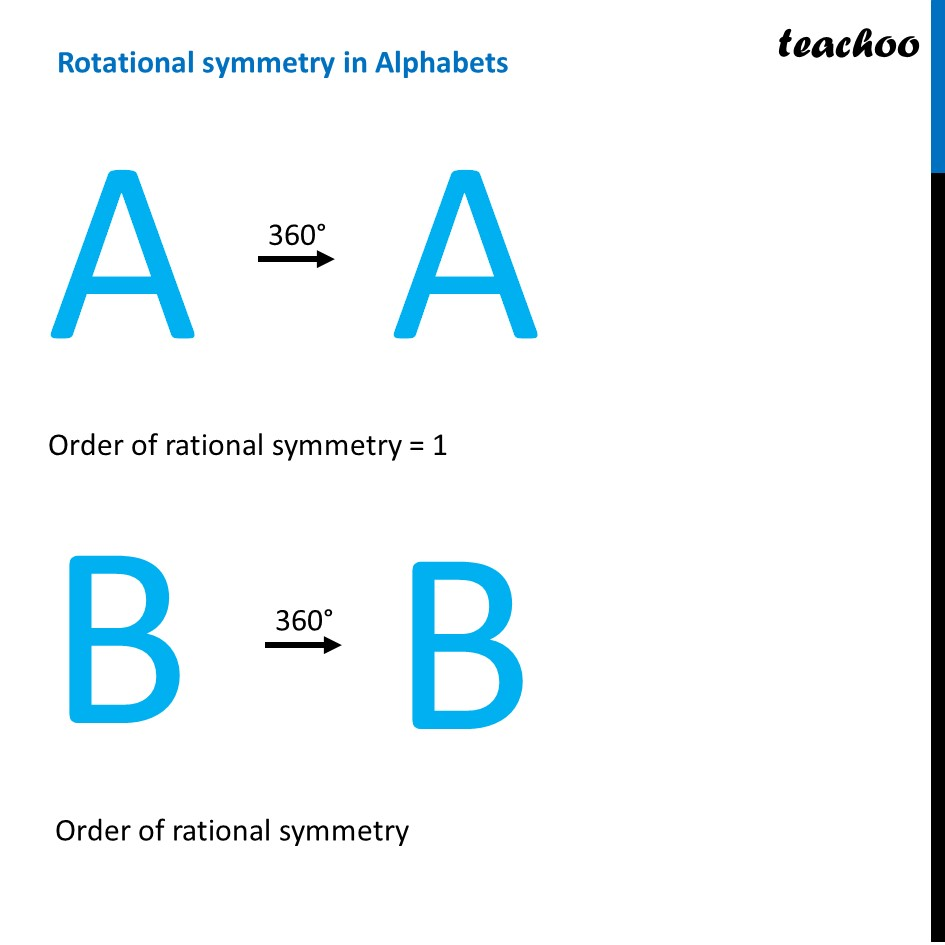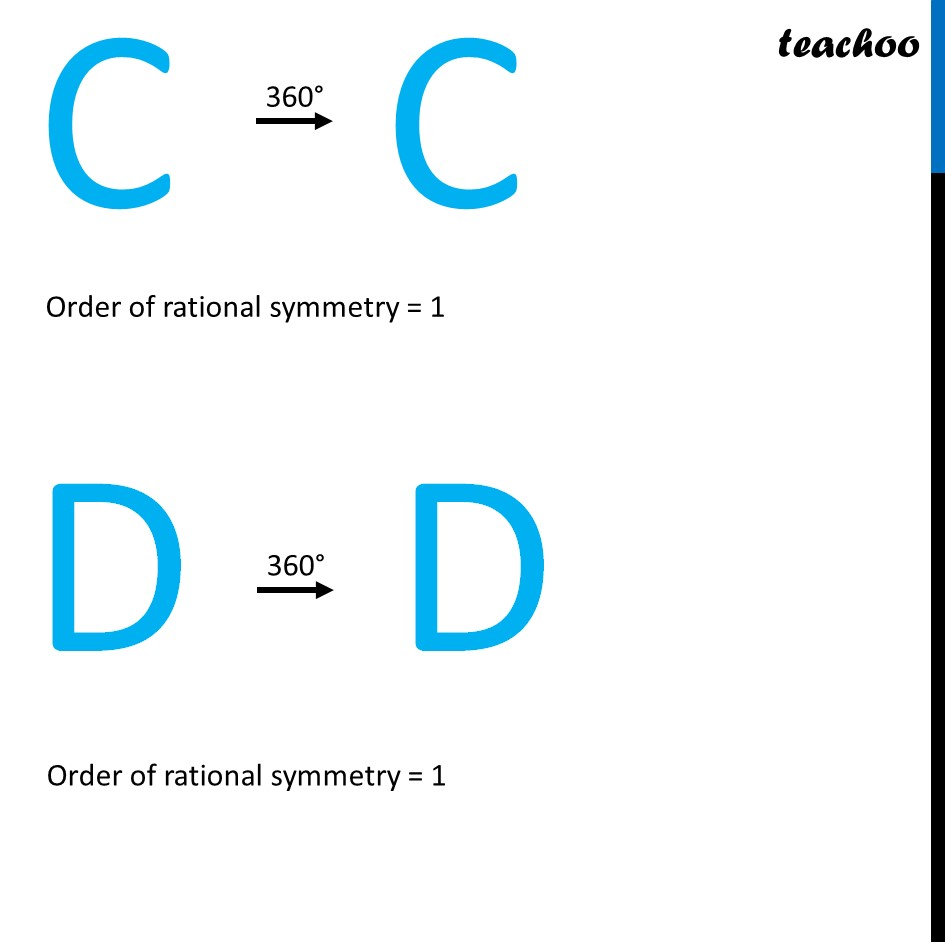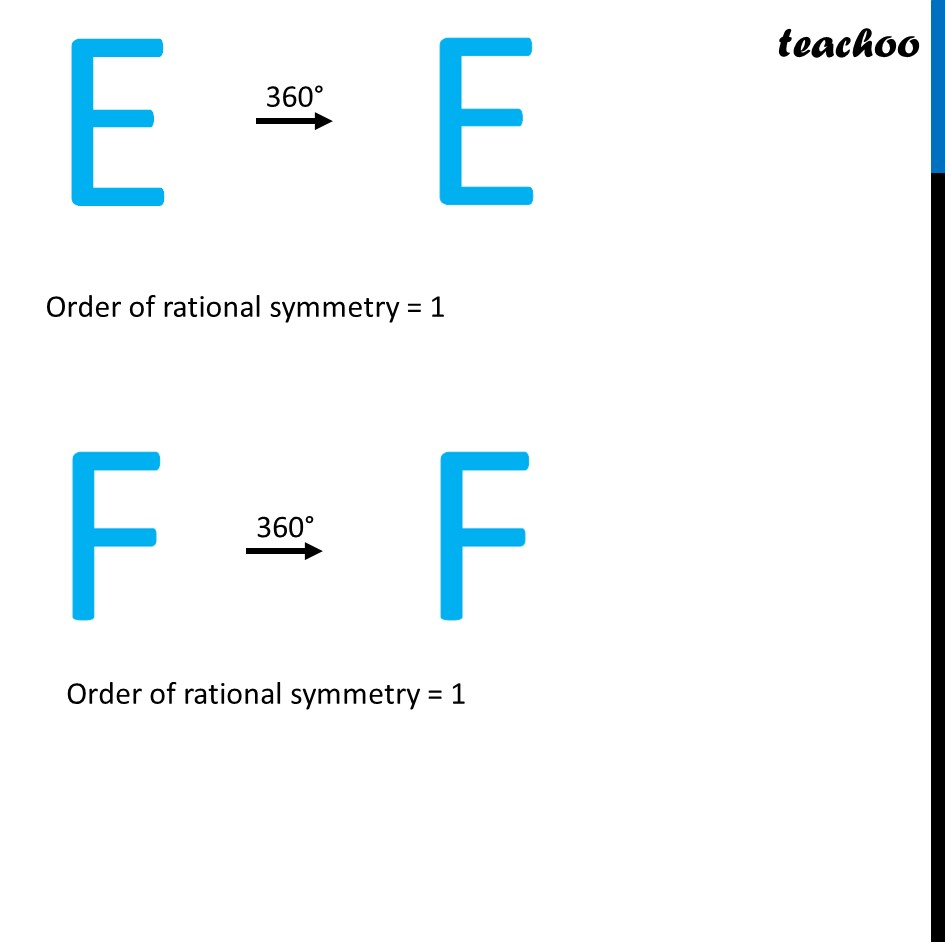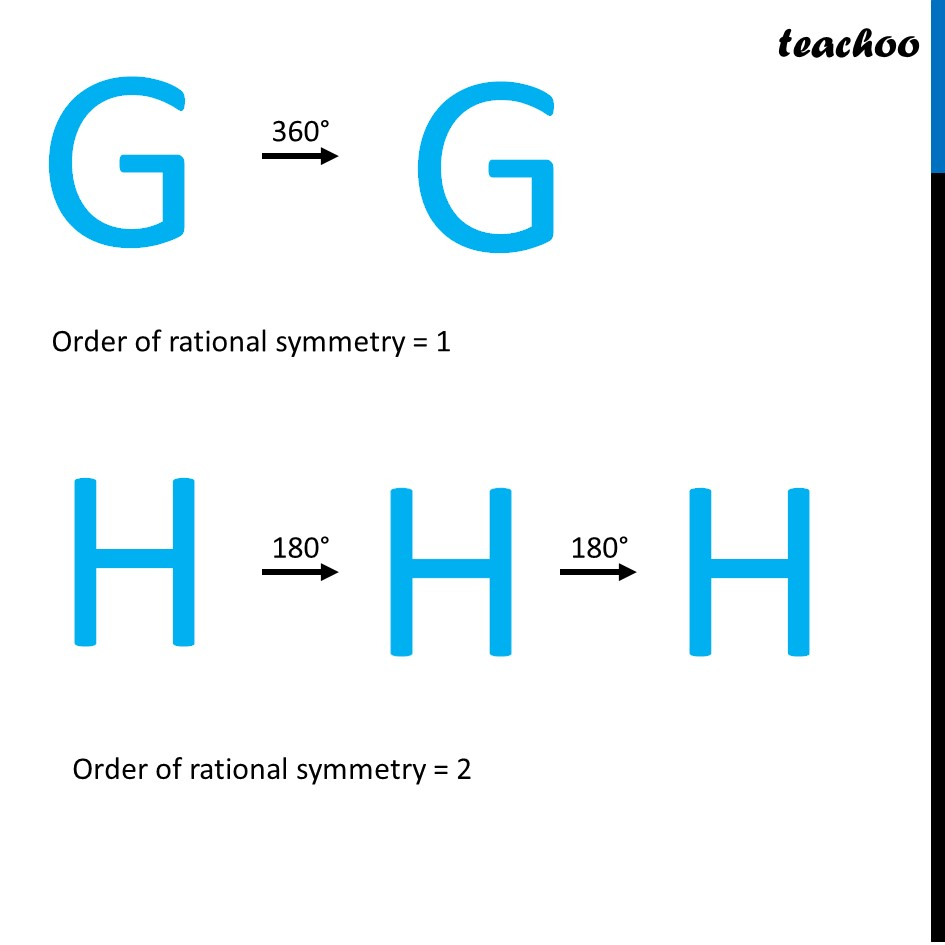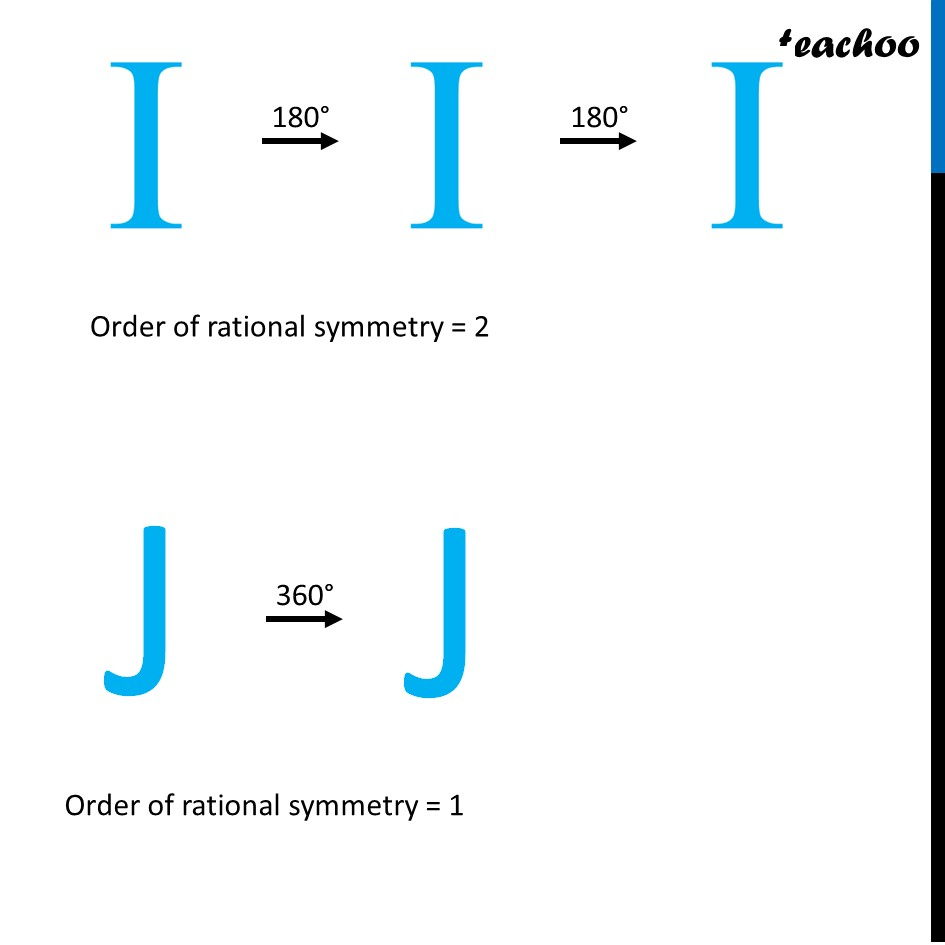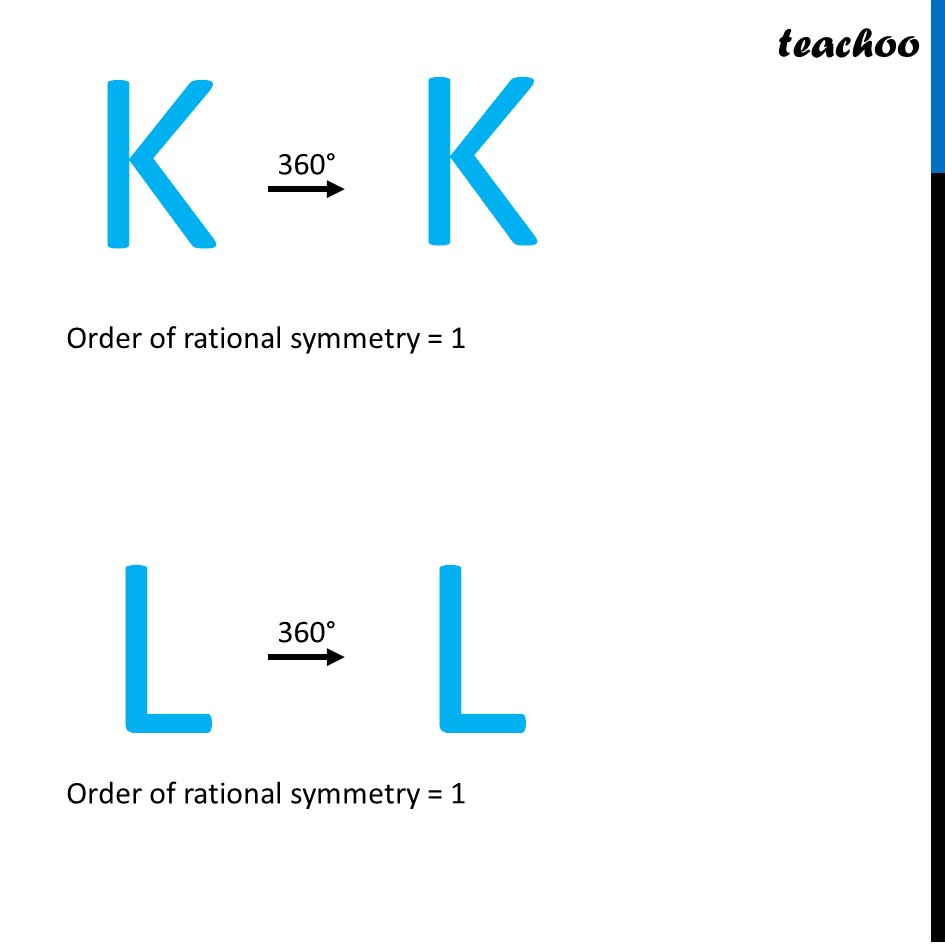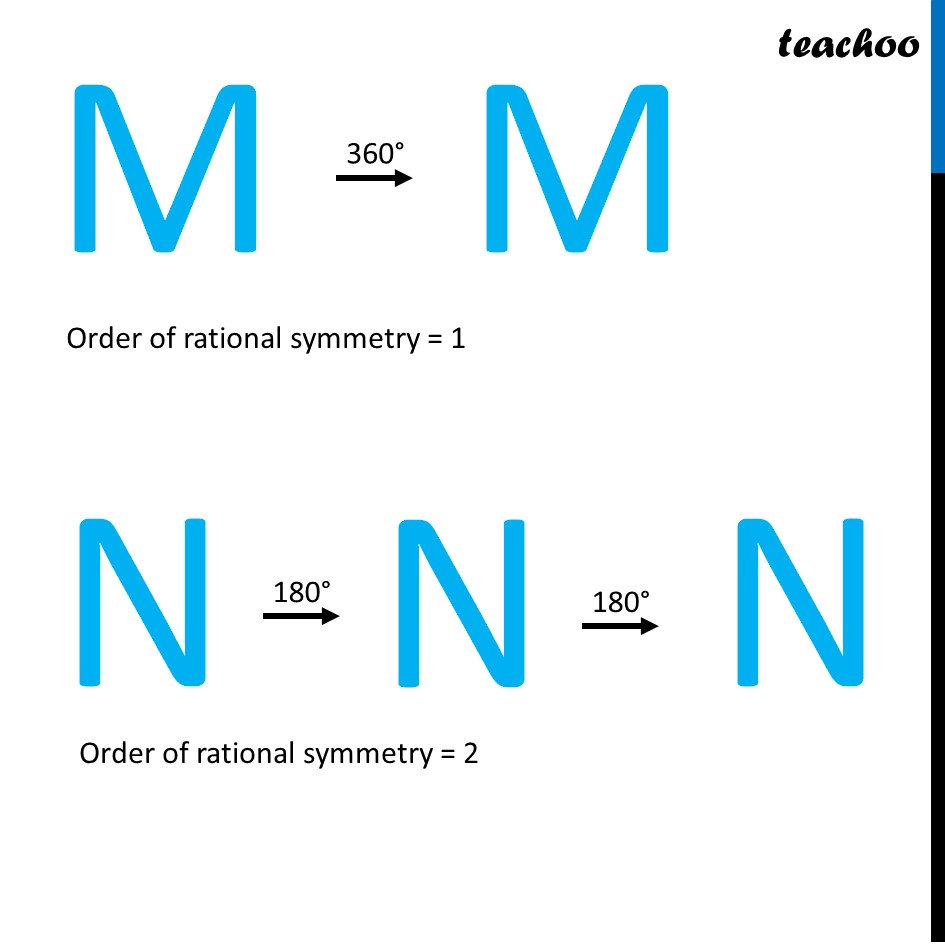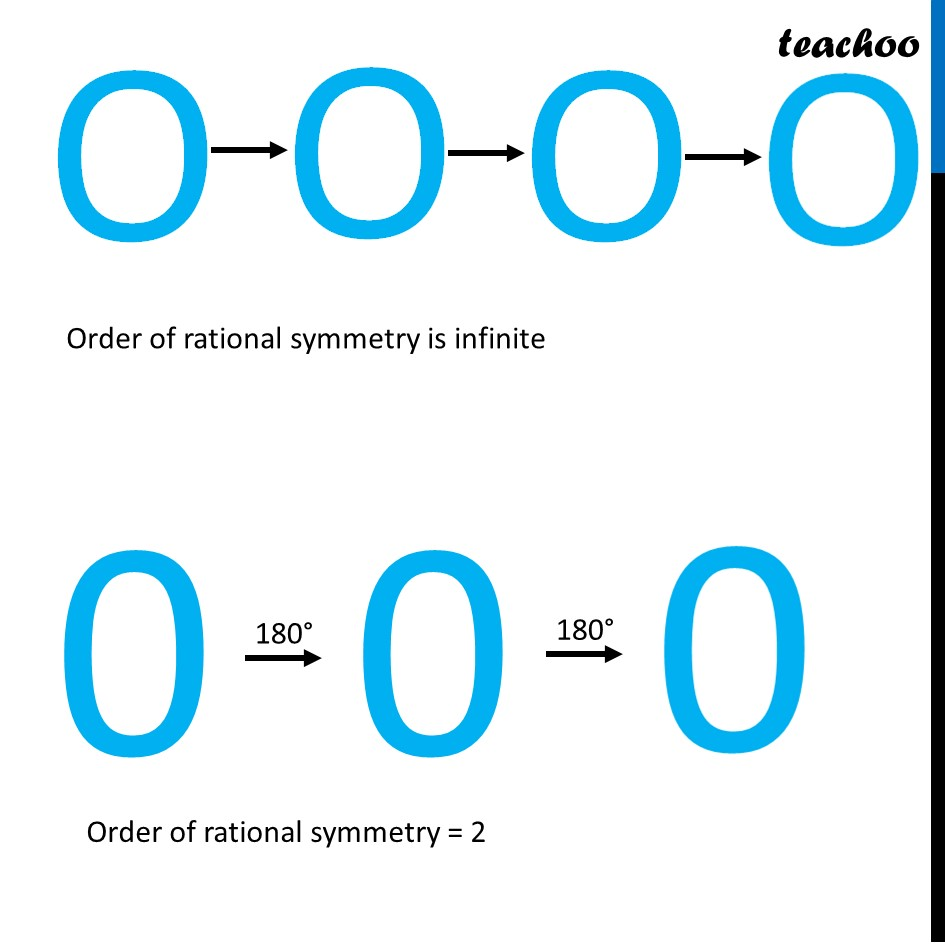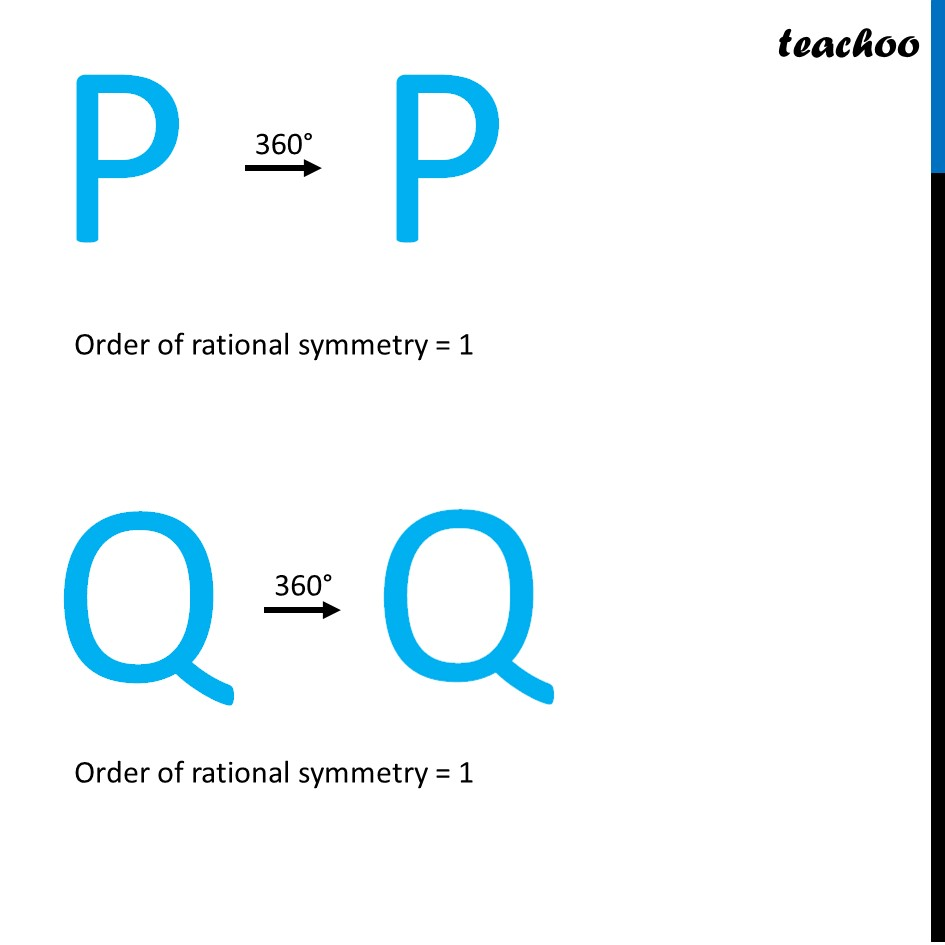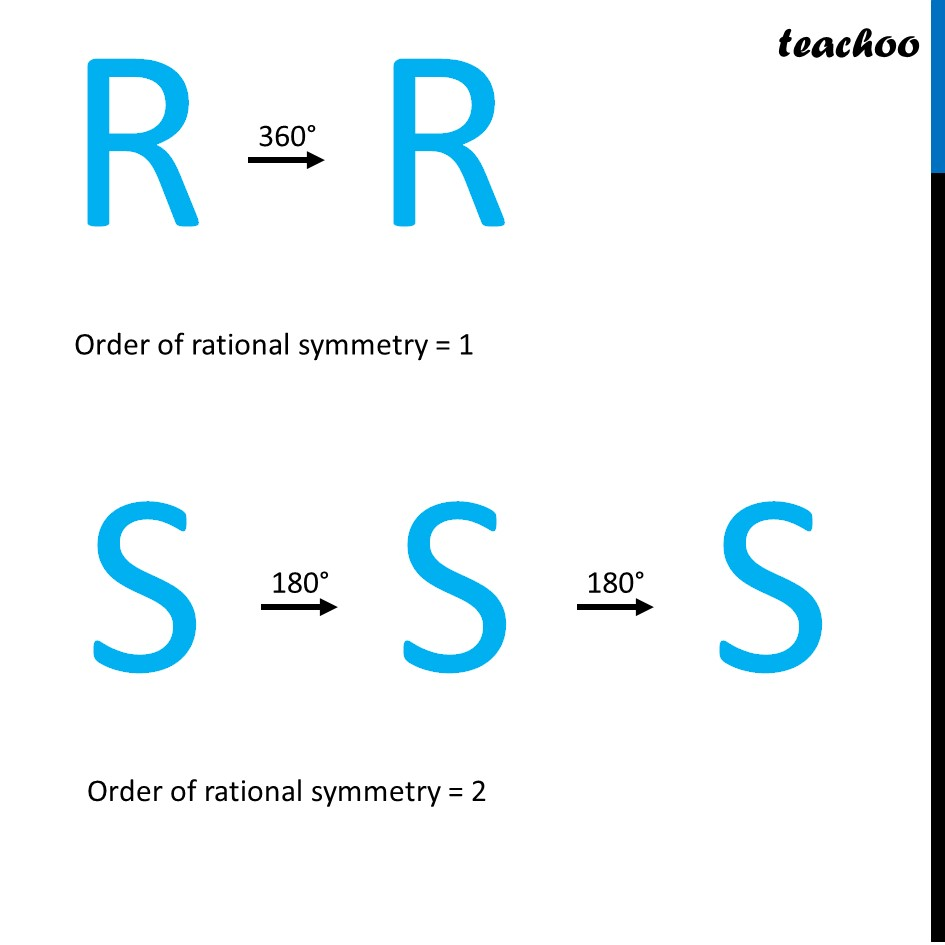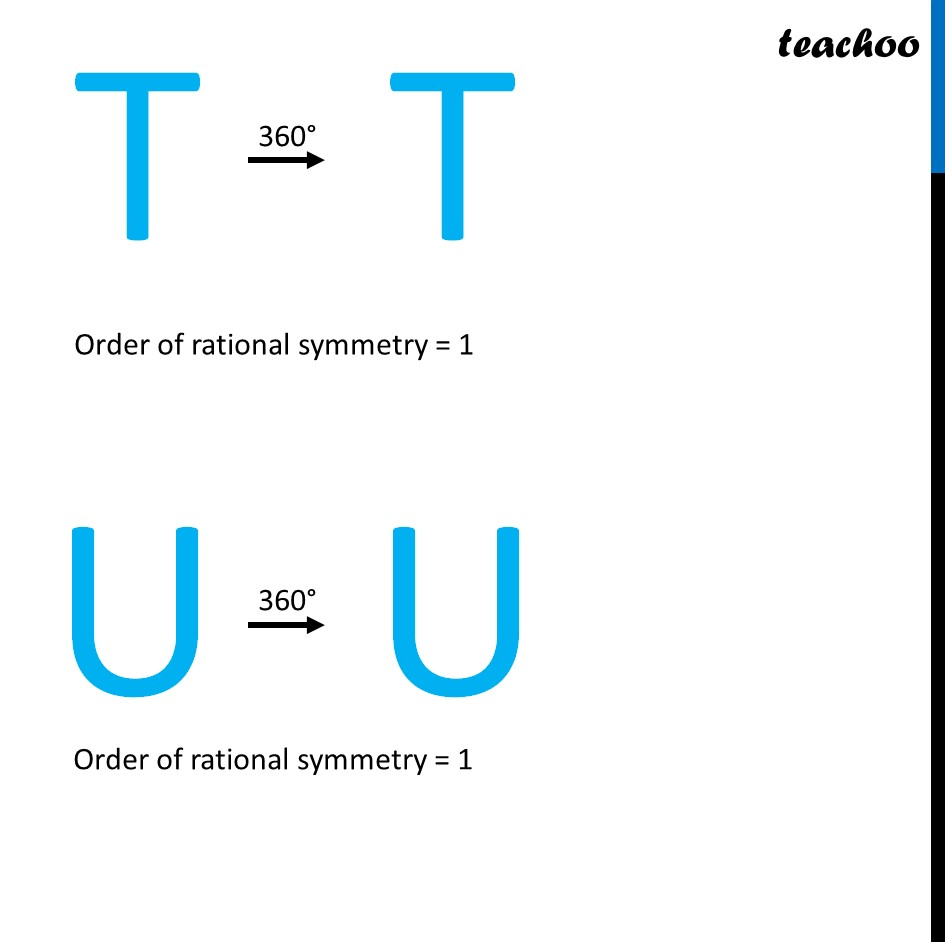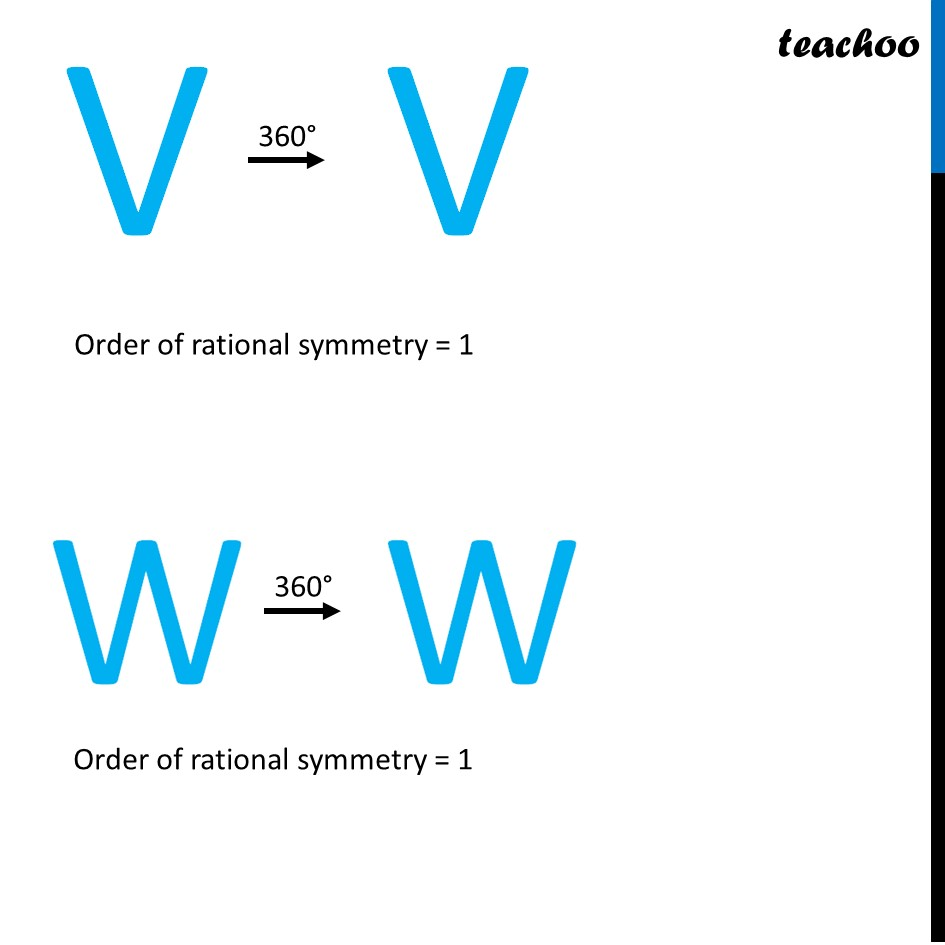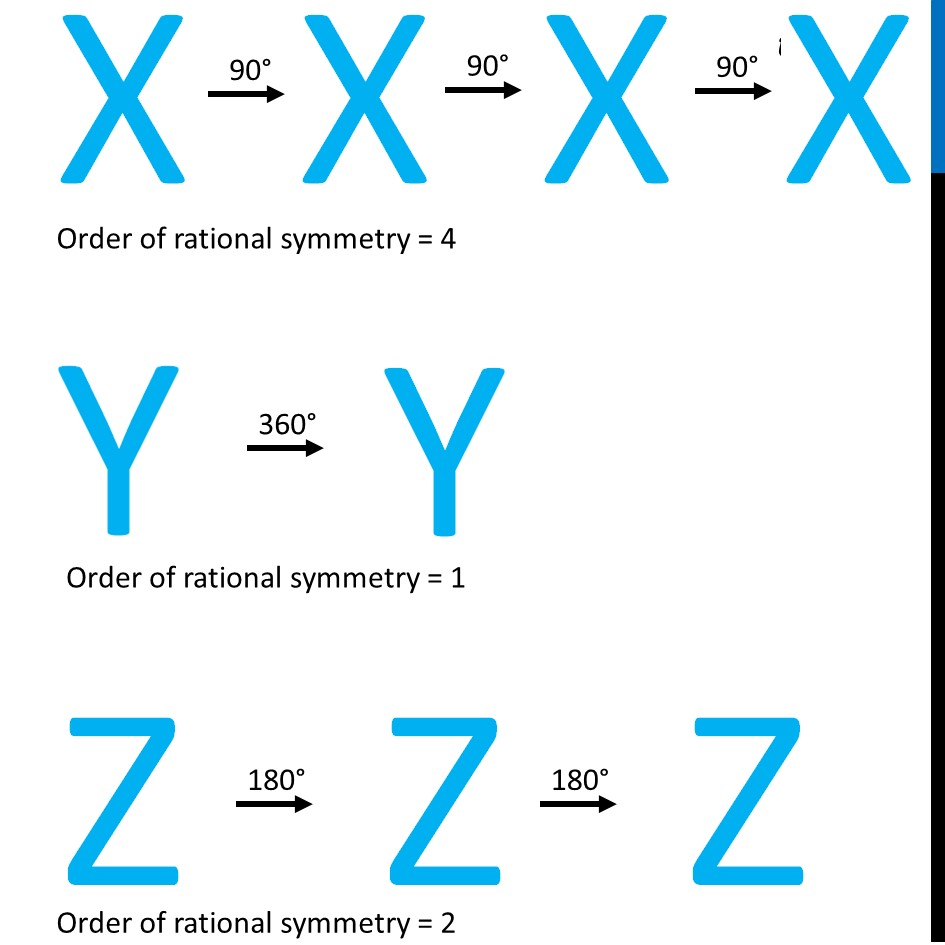Learn in your speed, with individual attention - Teachoo Maths 1-on-1 Class

### Transcript

Rotational symmetry in AlphabetsOrder of rational symmetry = 1 Order of rational symmetry Order of rational symmetry = 1 Order of rational symmetry = 1 Order of rational symmetry = 1 Order of rational symmetry = 1 Order of rational symmetry = 1 Order of rational symmetry = 2 Order of rational symmetry = 2 Order of rational symmetry = 1 Order of rational symmetry = 1 Order of rational symmetry = 1 Order of rational symmetry = 1 Order of rational symmetry = 2 Order of rational symmetry is infinite Order of rational symmetry = 2 Order of rational symmetry = 1 Order of rational symmetry = 1 Order of rational symmetry = 1 Order of rational symmetry = 2 Order of rational symmetry = 1 Order of rational symmetry = 1 Order of rational symmetry = 1 Order of rational symmetry = 1 Order of rational symmetry = 4 Order of rational symmetry = 1 Order of rational symmetry = 2